## Chapter Goals

• To recognize the correct ordering of decisions in multiple branches
• To program conditions using Boolean operators and variables
• To understand nested branches and loops
• To be able to program loops with the for and do/while statements
• To learn how to process character, word, and line input
• To learn how to read input from a file through redirection
• To implement approximations and simulations

## Multiple Alternatives

• By using collections of if/else statements, a program can distinguish between multiple alternatives.
• Example: The user enters the name of a coin, and the program returns the value.
• This program has five alternatives to choose from:
• "penny"
• "nickel"
• "dime"
• "quarter"
• erroneous input
• The order that the alternatives are checked is unimportant

## Multiple Alternatives (Coins Flowchart)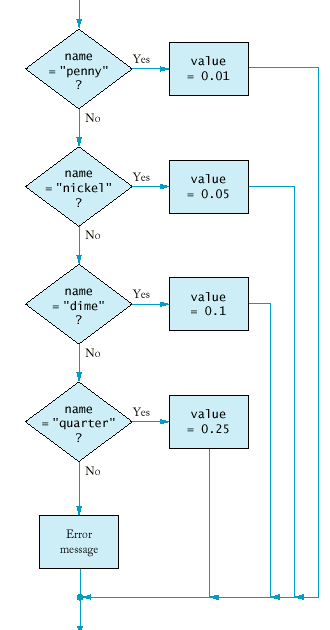## Multiple Alternatives

• In some cases, the order of the tests is important.
• Example: A program that displays a description of the likely impact of an earthquake based on its magnitude on the Richter scale.
• Note that the order of the tests ensures that the right results are printed (see following code).
• Note that the if/else/else structure ensure the alternatives are exclusive. Independent if statements may cause a single input to print several messages.

## Nested Branches

• Nested if/else statements can be used when there are two (or more) levels of decision making.
• Example: Taxes
• There is a different table for each marital status (decision 1).
• Once you have found the right table, your are taxed differently according to your income (decision 2).
•  If your status is Single and if the taxable income is over but not over the tax is of the amount over \$0 \$21,450 15% \$0 \$21,450 \$51,900 \$3,217.50 + 28% \$21,450 \$51,900 \$11,743 + 31% \$51,900

 If your status is Married and if the taxable income is over but not over the tax is of the amount over \$0 \$35,800 15% \$0 \$35,800 \$86,500 \$5,370.00 + 28% \$35,800 \$86,500 \$19,566.00+ 31% \$86,500
The two-level decision process is reflected in two levels of if statements.
• We say the income test is nested inside the test for filing status.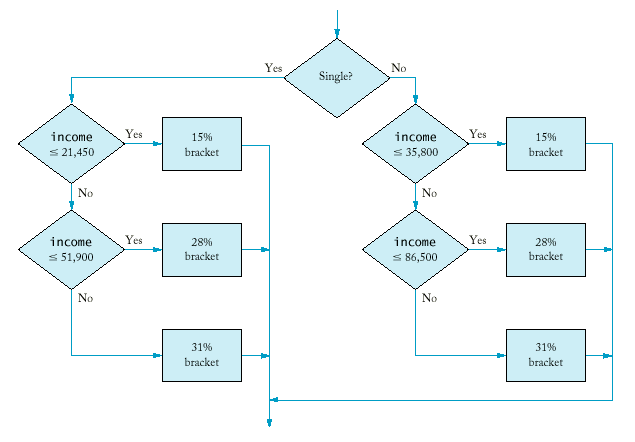• More complicated decisions may require deeper levels of nesting.

## Boolean Operations

• An operator that combines test conditions is called a logical operator.
• The && (and) operator combines several tests into a new test that passes only when all the conditions are true.
`if (now.get_hours() == homework.get_hours() &&    now.get_minutes() == homework.get_minutes())        cout << "The homework is due right now!\n";`
• The || (or) operator combines two or more conditions and succeeds if at least one of the conditions is true.
`if (state == "HI" || state == "AK")                     shipping_charge = 10.00;`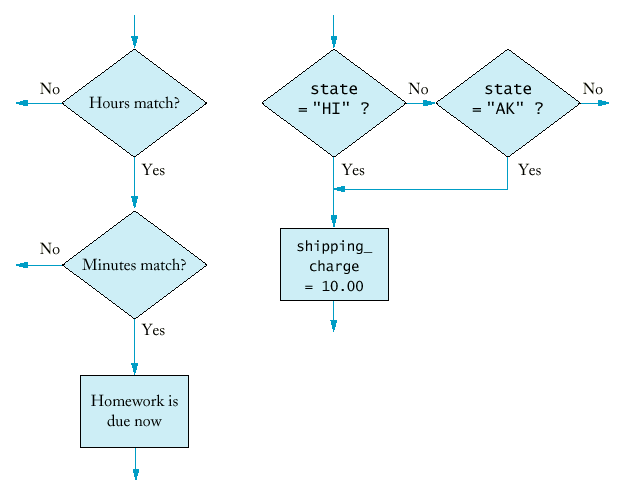The && and || operators are computed using lazy evaluation.
• The expressions are evaluated from left to right, and evaluation stops as soon as the truth value is determined.
`if (cin.fail() || area < 0) cout << "Input error.\n";	if (r >= 0 && -b / 2 + sqrt(r) >= 0) ...`
• The ! (not) operator takes a single condition and evaluates to true if that condition is false and to false if that condition is true.
`if (!cin.fail()) quarters = quarters + n;`
• Summary:

 A B A && B true true true true false false false Any false

 A B A ||B true Any true true false true false false false

 A !A true false false true

## DeMorgan's Law

• Humans generally have a hard time comprehending logical conditions with not operators applied to and/or expressions. Suppose we want to charge a higher shipping rate if we don't ship within the continental United States.
`if (!(country == "USA" && state != "AK" && state != "HI"))                      shipping_charge = 20.00;`
• DeMorgan's Law can be used to simplify these Boolean expressions.
• DeMorgan's Law has two forms:

 not (A and B) is the same as not A or not B not (A or B) is the same as not A and not B
• Pay particular attention to the fact that the and and or operators are reversed by moving the not inwards.
• Example:
`state != "AK" && state != "HI"`
means
`!(state == "AK") && !(state == "HI")`
Applying DeMorgan's Law to the original expression yields
`!(state == "AK" || state == "HI")`

## The for Loop

• The most common loop has the form
`i = start;while (i <= end){  . . .    i++;}`
• Because this loop is so common, there is a special form for it.
`for(i = start; i <= end; i++)`
• Example: Let us compute the function factorial, n! = 1.2.3.4...n.
`int product = 1;for (int i = 1; i <= n; i++) product = product * i;`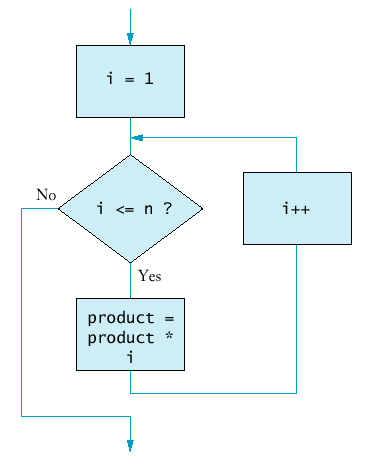## The for Loop (Syntax 7.1: for Statement)

Syntax 7.1 : for Statement

`for (initialization_expression; condition; update_expression) statement`
 Example: `for (int i = 1; i <= 10; i++) sum = sum + i;` Purpose: Execute the initialization statement. While the condition remains true, execute the statement and update the expression.

## The do Loop

• Sometimes you want to execute the body of a loop at least once and perform the loop test after the body was executed.
• The do/while loop serves that purpose.
`do{ statements}while (condition);`
• Example: A simple approximation algorithm to compute square root (ancient Greeks) - the sequence
x0 = xxn+1 = ( xn + x/xn ) / 2
converges and has limit square root of x.
• `do{  xold = xnew;   xnew = (xold + a / xold) / 2;}while (fabs(xnew - xold) > EPSILON);`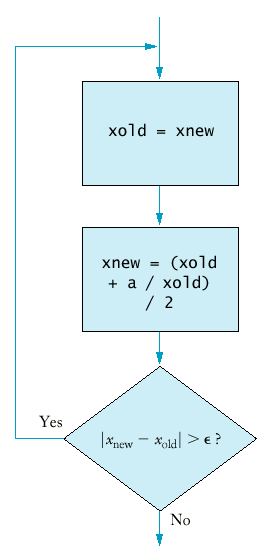## The do Loop (Syntax 7.2: do/while Statement)

Syntax 7.2: do/while Statement

`do statement while (condition);`
 Example: `do x = sqrt(x); while (x >= 10);` Purpose: Execute the statement, then test the condition, and repeat the statement while the condition remains true.

## Nested Loops

• How can we print a table of values?
• For example, this table tells you the fate of \$10,000 invested under various interest rates for a different number of years.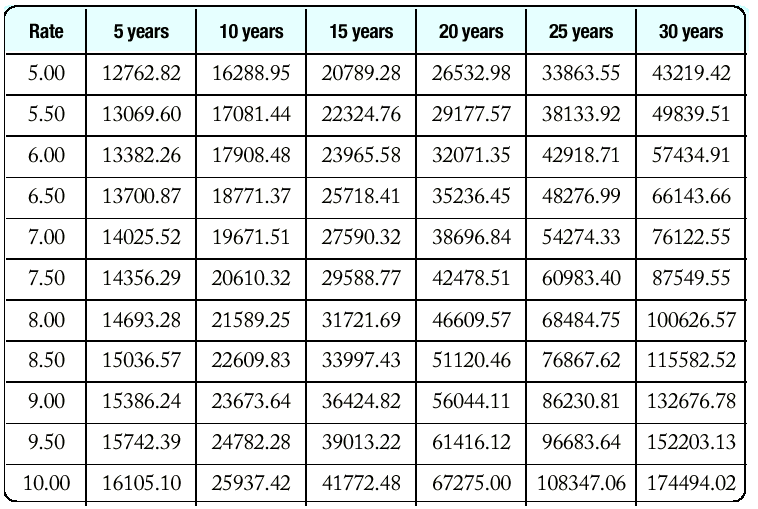• Here is the pseudocode:
`print table headerdouble rate;for (rate = RATE_MIN; rate <= RATE_MAX;      rate = rate + RATE_INCR){  print table row}`
• How do we print a table row? You need to program another loop.
`int year;for (year = YEAR_MIN; year <= YEAR_MAX;     year = year + YEAR_INCR){  balance = future_value(initial_balance, rate, year);   cout << setw(10) << balance;}`
• It's a good idea to print the table header as a loop as well.
`cout << "Rate     ";int year;for (year = YEAR_MIN; year <= YEAR_MAX;     year = year + YEAR_INCR){  cout << setw(2) << year << " years";}`
• Once everything is put together, you have the loop printing a single row nested in the loop that traverses the interest rates.

## Nested Loops

• Sometimes the iteration count of the inner loop depends on the outer loop.
• Example: printing a triangle shape.
`[][][][][][][][][][]`
• To print n rows, use the loop.
`for (int i = 1; i <= n; i++){  print triangle row}`
• Each row contains i boxes.
`for (int j = 1; j <= i; j++) cout << "[]";cout << "\n";`
• Putting the two loops together yields
`for (int i = 1; i <= n; i++){  for (int j = 1; j <= i; j++) cout << "[]";   cout << "\n";}`

## Processing Text Input

• When processing text input, is the input structured as a sequence of characters, words, or lines?
• To input a sequence of word, use the loop
`string word;while (cin >> word){  process word}`
• cin >> word has the same value as cin - it is the same test as !cin.fail().
• To process input one line at a time, use the getline function.
`string line;while (getline(cin, line)){  process line}`
• As above, the getline function returns cin, so you can test that cin hasn't yet failed.
• To process input character by character, use the loop
`char ch;while (cin.get(ch)){  process ch}`

## Processing Text Input (words.cpp)

• You would type text in, and at the end of the input the program would tell you how many words you typed. (You would never want to use such a program!)
• The program makes a lot of sense if input is read from a file.
• To link a file to the input of a program you type
`words < article.txt`
on a command line. The input instructions no longer expect input from the keyboard but from the file article.txt.
• Input redirection on the command line.
• If you can redirect also output of this program you type
`words < article.txt > output.txt`

## Simulations

• The C++ library has a random number generator, which produces numbers that appear to be random.
• Calling rand() yields a random number between 0 and RAND_MAX (usually 32767, but implementation dependent).
• These numbers actual come from a a very long sequence of numbers computed from fairly simple formulas; they just behave like random numbers.
• For that reason they are often called pseudorandom numbers.
• The following program produces the exact same output every time the program is run (because the numbers are generated with formulas).
`int main(){  int i;   for (i = 1; i <= 10; i++)   {  int r = rand();      cout << r << "\n";   }   return 0;}`
• When you specify a new seed for the random number sequence, the generator generates a new (different) sequence.
• The seed is set with the srand function.
• By setting the seed based on the time, the random numbers will be different every time you run the program.
`Time now;int seed = now.seconds_from(Time(0,0,0));srand(seed);`
• In actual applications you want to generate random numbers in different ranges, say between a and b.
• For example, to simulate a die roll, you need numbers between a = 1 and b = 6.
• First compute rand() % (b - a + 1) to obtain a random value between 0 and b - a.
• Add that to a to yield a random value between a and b.
`int rand_int(int a, int b){  return a + rand() % (b - a + 1);}`

## Simulations

• To generate a random floating-point number, note that the quantity rand() * 1.0 / RAND_MAX is a random floating-point value between 0 and 1.
• The 1.0 ensures that the division is a floating-point number.
`double rand_double(double a, double b){  return a + (b - a) * rand() * (1.0 / RAND_MAX);}`

## Simulations (Buffon Needle Experiment)

• Devised by Comte George-Louis Leclerc de Buffon (1707-1788), a French naturalist.
• A needle of length 1 inch is dropped onto paper that is ruled with parallel lines 2 inches apart.
• If the needle drops onto a line, we count it as a hit.
• The quotient tries / hits approximates pi.
• Our program randomly generates the lower point of the needle and an angle.
• The upper point of the needle is given by yhigh = ylow + sin(angle).
• If yhigh >= 2, we count the needle as a hit.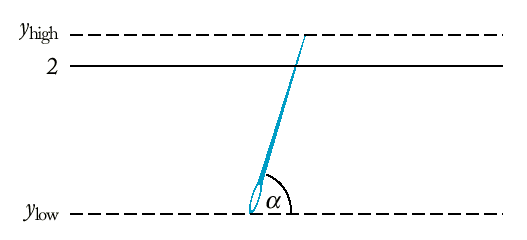## Simulations (buffon.cpp)

The point of this program is not to compute pi (after all, we needed the value of pi in the deg2rad function). Rather, the point is to show how to a physical experiment can be simulated on the computer.